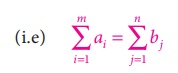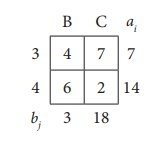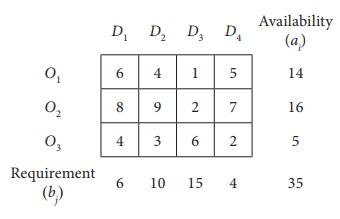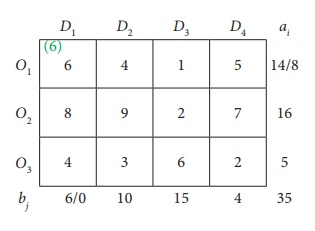Home | | Business Maths 12th Std | Methods of finding initial Basic Feasible Solutions: North-West Corner Rule (NWC)

# Methods of finding initial Basic Feasible Solutions: North-West Corner Rule (NWC)

Operations Research: Transportation Problem: Methods of finding initial Basic Feasible Solutions: North-West Corner Rule (NWC)

Methods of finding initial Basic Feasible Solutions

There are several methods available to obtain an initial basic feasible solution of a transportation problem. We discuss here only the following three. For finding the initial basic feasible solution total supply must be equal to total demand.## Method: North-West Corner Rule (NWC)

It is a simple method to obtain an initial basic feasible solution. Various steps involved in this method are summarized below.

Step 1: Choose the cell in the north- west corner of the transportation Table10.1 and allocate as much as possible in this cell so that either the capacity of first row (supply)is exhausted or the destination requirement of the first column(demand) is exhausted. (i.e) x11 = min(a1,b1)

Step 2: If the demand is exhausted (b1 < a1), move one cell right horizontally tothe second column and allocate as much as possible.(i.e) x12= min (a1 ŌĆōx11b2)

If the supply is exhausted (b1 > a1), move one cell down vertically to the second row and allocateas much as possible.(i.e)x21 = min (a2,b1 ŌĆō x11)

If both supply and demand are exhausted move one cell diagonally and allocate as much as possible.

Step 3: Continue the above procedure until all the allocations are made

Example 10.1

Obtain the initial solution for the following problemSolution:

Here total supply = 5+8+7+14=34, Total demand = 7+9+18=34

(i.e) Total supply =Total demandŌł┤The given problem is balanced transportation problem.

Ōł┤ we can findan initial basic feasible solution to the given problem.

From the above table we can choose the cell in the North West Corner. Here the cell is (1,A)

Allocate as much as possible in this cell so that either the capacity of first row is exhausted or the destination requirement of the first column is exhausted.

i.e. x11 = min (5,7) = 5Reduced transportation table isNow the cell in the North west corner is (2, A)

Allocate as much as possible in the first cell so that either the capacity of second row is exhausted or the destination requirement of the first column is exhausted.

i.e. x12 = min (2,8) = 2Reduced transportation table isHere north west corner cell is (2,B) Allocate as much as possible in the first cell so that either the capacity of second row is exhausted or the destination requirement of the second column is exhausted.

i.e. x22 = min (6,9) = 6Reduced transportation table isHere north west corner cell is (3,B).

Allocate as much as possible in the first cell so that either the capacity of third row is exhausted or the destination requirement of the second column is exhausted.

i.e. x32 = min (7,3) = 3Reduced transportation table isHere north west corner cell is (3,C) Allocate as much as possible in the first cell so that either the capacity of third row is exhausted or the destination requirement of the third column is exhausted.

i.e. x33 = min (4,18) = 4Reduced transportation table and final allocation is x44 = 14Thus we have the following allocationsTransportation schedule : 1ŌåÆ A, 2ŌåÆA, 2ŌåÆB, 3ŌåÆB, 3ŌåÆC, 4ŌåÆC

The total transportation cost.

= (5 ├Ś 2) + (2 ├Ś 3) +(6 ├Ś 3)+(3 ├Ś 4)+(4 ├Ś 7) + (14 ├Ś 2)

= Rs.102

Example 10.2

Determine an initial basic feasible solution to the following transportation problem using North West corner rule.Here Oi and Dj represent ith origin and jth destination.

Solution:

Given transportation table isTotal Availability = Total Requirement

Ōł┤ The given problem is balanced transportation problem.

Hence there exists a feasible solution to the given problem.

First allocation:Second allocation:Third Allocation:Fourth Allocation:Fifth allocation:Final allocation:Transportation schedule : O1ŌåÆ D1, O1ŌåÆD2, O2ŌåÆD2, O2ŌåÆD3, O3ŌåÆD3, O3ŌåÆD3.

The transportation cost

= (6 ├Ś 6)+(8 ├Ś 4)+(2 ├Ś 9)+(14 ├Ś 2)+(1 ├Ś 6)+(4 ├Ś 2) =Rs.128

Tags : Solved Example Problems | Transportation Problem | Operations Research , 12th Business Maths and Statistics : Chapter 10 : Operations Research
Study Material, Lecturing Notes, Assignment, Reference, Wiki description explanation, brief detail
12th Business Maths and Statistics : Chapter 10 : Operations Research : Methods of finding initial Basic Feasible Solutions: North-West Corner Rule (NWC) | Solved Example Problems | Transportation Problem | Operations Research